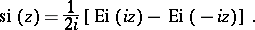# Integral sine

(diff) ← Older revision | Latest revision (diff) | Newer revision → (diff)

The special function defined for realbyFor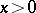one has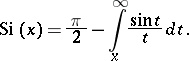One sometimes uses the notation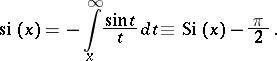Some particular values are: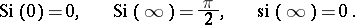Some special relations: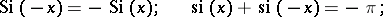where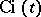is the integral cosine. Forsmall,The asymptotic representation for largeis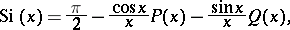whereThe integral sine has the series representation(*)

As a function of the complex variable,, defined by (*), is an entire function ofin the-plane.

The integral sine is related to the integral exponential function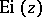by